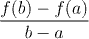Analysis WebNotes
arrow_back When you work out the slope of the straight line joining (a,f(a)) to (b,f(b)), it comes out to beIt's no surpise that this is the quantity from the statement of the theorem, because that's really exactly what the mean value theorem says:

Provided f is suitably continuous and differentiable, there is a point in (a,b) at which the instantaneous slope of f is the same as the slope of the straight line joining the values of f at a and b.